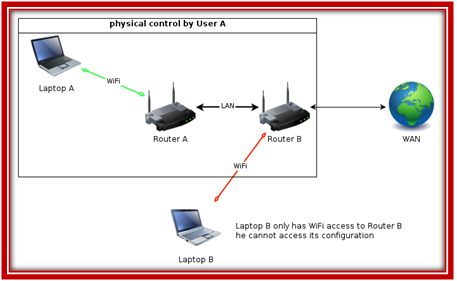# NS3 Wifi Projects

## NS3 Wifi Projects

Wi-Fi is the name given by the Wi-Fi Alliance to the IEEE 802.11 suite of standards. 802.11 defined the initial standard for wireless local area networks (WLANs), but it was considered too slow for some applications and so was superseded by the extensions 802.11a and 802.11b, and later by 802.11gBenefits of NS3 WIFI Projects:

• It offers extended access, cost reduction and mobility create opportunities for new applications as well as the possibility of creative new solutions for legacy applications
• Cost reduction.
• Extended access.
• It’s an Ethernet placement.
• Gives flexibility.

Characteristics of WIFI:

• Signal strength and range.
• Speed needs.
• Operating frequency.
• Channels .
• Data rate throughputs.

#### Ns3 Video Tutorial

Ns3 Video Tutorial – Latest NS3 Projects Output

#### Ns3 Tutorial

Ns3 Tutorial – Guide to Various Network Projects

#### Journal Support

Paper Publication for NS3 Simulation Projects

#### Contact Form

Sample code for NS3 WIFI PROJECTS :
This is the sample code of NS3 WIFI NETWORK PROJECTS in distance calculation and range setting.
static void PrintPsrVsDistance (int argc, char *argv[])
{
struct PsrExperiment::Input input;
CommandLine cmd;
cmd.AddValue (“TxPowerLevel”, “The power level index to use to send each packet”, input.txPowerLevel);
cmd.AddValue (“TxMode”, “The mode to use to send each packet”, input.txMode);
cmd.AddValue (“NPackets”, “The number of packets to send”, input.nPackets);
cmd.AddValue (“PacketSize”, “The size of each packet sent”, input.packetSize);
cmd.Parse (argc, argv);
for (input.distance = 1.0; input.distance < 165; input.distance += 2.0)
{
std::cout << input.distance;
PsrExperiment experiment;
struct PsrExperiment::Output output;

input.txMode = “OfdmRate6Mbps”;
output = experiment.Run (input);
std::cout << ” ” << CalcPsr (output, input);

input.txMode = “OfdmRate9Mbps”;
output = experiment.Run (input);
std::cout << ” ” << CalcPsr (output, input);

input.txMode = “OfdmRate12Mbps”;
output = experiment.Run (input);
std::cout << ” ” << CalcPsr (output, input);

input.txMode = “OfdmRate18Mbps”;
output = experiment.Run (input);
std::cout << ” ” << CalcPsr (output, input);

input.txMode = “OfdmRate24Mbps”;
output = experiment.Run (input);
std::cout << ” ” << CalcPsr (output, input);

input.txMode = “OfdmRate36Mbps”;
output = experiment.Run (input);
std::cout << ” ” << CalcPsr (output, input);

input.txMode = “OfdmRate48Mbps”;
output = experiment.Run (input);
std::cout << ” ” << CalcPsr (output, input);

input.txMode = “OfdmRate54Mbps”;
output = experiment.Run (input);
std::cout << ” ” << CalcPsr (output, input);

std::cout << std::endl;
}
}

static void PrintSizeVsRange (int argc, char *argv[])
{
double targetPsr = 0.05;
struct PsrExperiment::Input input;
CommandLine cmd;
cmd.AddValue (“TxPowerLevel”, “The power level index to use to send each packet”, input.txPowerLevel);
cmd.AddValue (“TxMode”, “The mode to use to send each packet”, input.txMode);
cmd.AddValue (“NPackets”, “The number of packets to send”, input.nPackets);
cmd.AddValue (“TargetPsr”, “The psr needed to assume that we are within range”, targetPsr);
cmd.Parse (argc, argv);
for (input.packetSize = 10; input.packetSize < 3000; input.packetSize += 40) { double precision = 0.1; double low = 1.0; double high = 200.0; while (high – low > precision)
{
double middle = low + (high – low) / 2;
struct PsrExperiment::Output output;
PsrExperiment experiment;
input.distance = middle;
output = experiment.Run (input);
double psr = CalcPsr (output, input);
if (psr >= targetPsr)
{
low = middle;
}
else
{
high = middle;
}
}
std::cout << input.packetSize << ” ” << input.distance << std::endl;
}
}